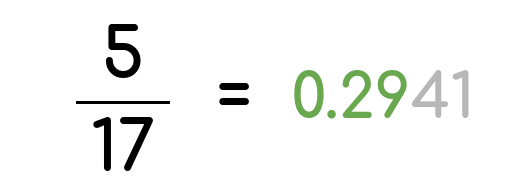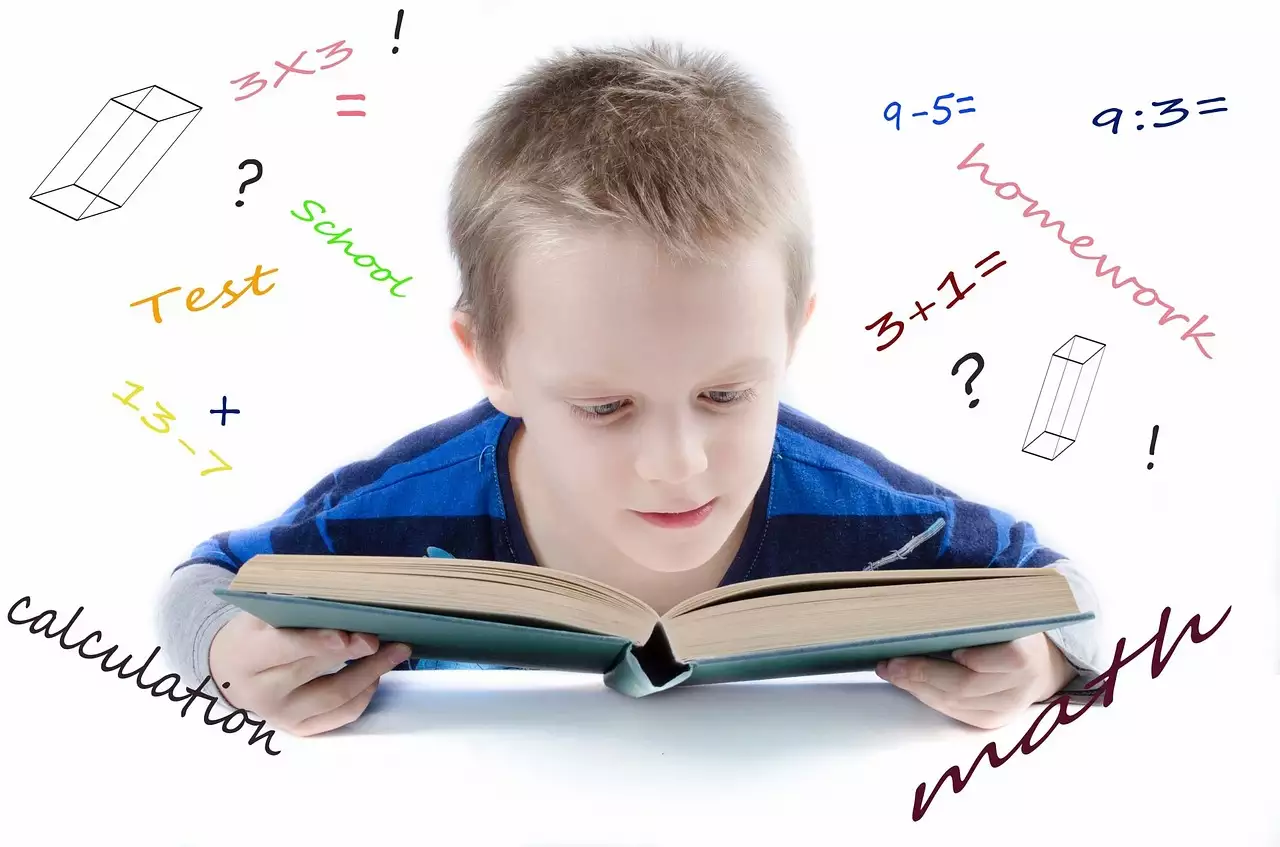# Rounding Fractions calculator

## Learn how to round even complex fractions using various approximation methodsThe rounding fractions calculator doesn’t only turn fractions into the nearest whole number to use in your calculations. It can also round to various decimal precisions.

All you need to do is input the fraction’s numerator and denominator into the tool. Then you can select the required rounding precision and click “calculate” to get the results.

## How to Round Fractions?

There are a whole lot of ways to round numbers, including ceiling, floor, half-up, and half-down. That said, the half-up is the most widely accepted one.

Here’s how this rounding method works:

1. Convert the fraction to a decimal.
2. Check the value of the first decimal place.
3. If it’s lower than 5, ignore it and everything that’s on its right.
4. If it’s higher than or equal to 5, add 1 to the digit on the left and remove everything else (including the rounded decimal.)

Let’s see how you can use the half-up rounding method with a few examples.

Suppose the fraction you’re working with is 23/5. If you divide the numerator by the denominator, you’ll get 4.6. According to the half-up method, you can round that 4.6 to 5 since 6 is higher than the half-point.

Similarly, if you’re working with 23/7, you’ll get 3.28571428571. Now, that’s a rather chunky and intimidating number. However, the same half-up rule applies; since the 2 in the first decimal place is lower than 5, you can just say that 23/7 rounds up to 3.

But what would you do if the fraction you need to round is 23/2, which is exactly 11.5? Well, you’ll still round it up to the nearest neighbor, which is 12.

### Changing the Precision

In all the previous examples, we rounded to the nearest whole number. However, it’s also possible to choose a different precision from the following options:

• Thousandth (rounding to 3 decimals)
• Hundredth (rounding to 2 decimals)
• Tenth (rounding to 1 decimal)
• Integer
• Tens
• Hundreds
• Thousands
• Millions
• Billions

Take, for instance, the 23/7 fraction. Earlier, we rounded it to 3 from 3.28571428571, but that’s only because we wanted to round it to the nearest integer.

If you choose to round the tenth, then you’ll see that the 8 in the second decimal position is higher than the half-point. So, you can add 1 to the first decimal position and ignore everything else. As a result, 23/7 rounds up to 3.3.

On the other hand, if you want to round to the hundredth, the 23/7 fraction will turn out to be 3.29. That’s because the third decimal is 5, and you can add 1 to the second decimal place to shift it from 8 to 9.

Keep in mind that the CalcoPolis fraction rounding calculator is set to a precision of the hundredth by default. So, if you need to round to integers or any other accuracy level, make sure to adjust the setting in the “round to” drop list first.

### Rounding Negative Fractions

All the examples we’ve checked so far involved positive fractions, and in these cases, we rounded up toward positive infinity. This means that we opted for the highest neighbor whenever possible, but is that the case for negative values?

While dealing with negative fractions can be a bit confusing, picturing the fraction’s position on the number line helps.

Some people take the negative number closer to positive infinity. So, they might round the -23/2 fraction to -11 from -11.5.

Instead, you’ll need to go towards negative infinity and round the same fraction to -12. That’s the result that you can expect when you’re using the CalcoPolis fraction rounding calculator.

## Role of Rounding Fractions in EducationRounding fractions has always been an integral part of mathematical education. As students progress in their studies, they frequently encounter situations where they need to convert fractions to more manageable forms, such as decimal values or to the nearest whole number. By doing so, they can simplify complex problems and make their calculations quick and efficient.

One of the fundamental exercises often taught is rounding a fraction to the nearest hundredth. This method not only helps in simplifying the fraction but also lays the foundation for understanding decimal values. When students round fractions to the nearest hundredth, they develop a keen sense of precision, which is crucial in advanced mathematical studies and real-life applications.

Using tools like the rounding fractions calculator to round numbers has become increasingly popular in classrooms. These digital aids enable students to check their manual calculations and gain confidence in their rounding skills. It also allows them to experiment and see the effects of different rounding methods on various fractions.

Rounding to the nearest whole is another fundamental skill. When students focus on this method, they learn to identify the rounding digit and make decisions based on its value. For instance, when rounding 3/4 to the nearest whole, they determine whether it's closer to 0 or 1. This skill is especially useful when dealing with real-world scenarios where exact decimal values aren't necessary, and approximations can suffice.

So, rounding fractions in education is not just about simplifying numbers; it's about building a foundational understanding of numbers and their relationships. By mastering this skill early on, students are better equipped to handle more complex mathematical challenges in the future.

You can find many more helpful tools here at Calcopolis. For rounding money amounts, we recommend you to visit the rounding money amounts calculator.

If you wish to convert fractions to a simpler form, you may use our fraction simplifier calculator. The tool will give you fractions instead of rounded decimals.

For a complex fraction, you may use the mixed number to a decimal converter to reduce the mixed number to a simple fraction. Alternatively, you can convert a fraction to a percentage value.

### Authors

Created by Lucas Krysiak on 2022-11-21 16:53:53 | Last review by Mike Kozminsky on 2022-12-09 10:58:00